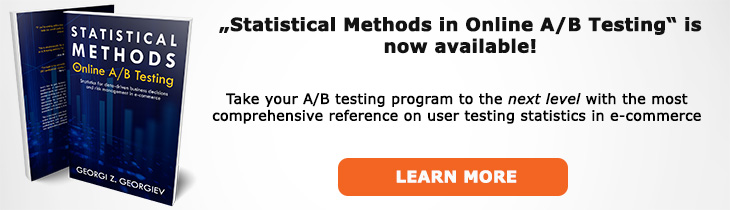# What does "Distribution" mean?

Definition of Distribution in the context of A/B testing (online controlled experiments).

## What is a Distribution?

In probability theory the distribution of a parameter is a description of the relative frequency with which each possible outcome would occur if an independent number of samples of this parameter are observed. A probability distribution in particular is a description of a random phenomenon in terms of the probabilities of events. It is usually plotted on an X/Y plot where the X axis contains particular values of the parameter (see sample space) while the Y axis shows the relative frequency.

A distribution might be discrete such as a distribution of the conversions per user or transactions per user in an A/B test or they might be continuous such as when describing a KPI such as average revenue per user. Generally the distribution of a binomial metric is discrete and that of a non-binomial metric is continuous.

Many distributions such as the normal distribution have known probability density functions (PDF) and cumulative distribution functions (CDF). The PDF describes the probability a given value will occur and it is evaluated at a given point. The CDF describes the cumulative probability that a given value or any value smaller than it will occur. alpha and the p-value are calculated using the cumulative probability function.

## Related A/B Testing terms

Sample Space

Normal Distribution

Like this glossary entry? For an in-depth and comprehensive reading on A/B testing stats, check out the book "Statistical Methods in Online A/B Testing" by the author of this glossary, Georgi Georgiev.## Glossary Index by Letter

Select a letter to see all A/B testing terms starting with that letter or visit the Glossary homepage to see all.